# 【Python 小白到精通 | 课程笔记】第三章：数据处理就像侦探游戏（函数和包）

### 文章目录

• 🚩 写在前面
• 划分学习内容
• 学到的一些操作（简单的罗列）
• 保留问题
• 🌵 课后作业
• 1、写出1960年GDP最高的国家：有一行是World不是国家
• 问题：Dataframe取索引，但列名含有空格
• 注意：Dataframe用来赋值返回的是一个引用
• 2、求多个年份GDP前三的国家
• 3、选择几个你感兴趣的国家，画合适的图表示他们在60年间的GDP变化
• 问题1：'list' object has no attribute 'plot'
• 问题2：画直方图，发现x、y轴反了
• 问题3：matplotlib绘图，坐标轴无法显示中文
• 4、自选问题：这60年间，GDP增长率最大的国家（或组织）是哪个？
• 🧭 经验总结
• 🧾 参考资料（仅部分）
• # 🚩 写在前面

Al Studio课程地址：第三章：数据处理就像侦探游戏（函数和包）

第三章的学习，我突然就开始感觉到吃力了。课程视频只有 20 来分钟，倒用不了多久就能看完。但当更加仔细地去阅读 markdown 讲义和源代码的时候，真的很消耗精力。

这章主要学习了：

• 数据处理流程。碰到异常的数据，得思考它是如何产生的，同时可以寻找一些外部信息的帮助。
• numpy
• pandas：Series（列），Dataframe（表格）
• Dataframe 的各种操作真的令我有些眼花缭乱了，好在我还是看完了，并在尝试修改源代码的过程中大致知道了它是怎么一回事儿。

# 划分学习内容

把看到的都记住显然是划不来的，本章内容较多，课程还对学习内容进行了分类，感觉这样挺好。# 学到的一些操作（简单的罗列）

1、读取csv文件

``````example_data = pd.read_csv('work/example.csv')
``````

2、按序列号索引名取记录：

``````print(titanic_data.iloc[0, 3])
print(titanic_data.loc[0, 'Age'])
``````

3、显示基本信息，像 column 名，空数据个数，数据类型：

``````titanic_data.info()
``````

4、有多条记录时可以只显示前几条

``````.head()
``````

5、填充数据

``````fillna('')
``````

6、对数据分类进行操作

``````print(titanic_data.groupby(['Pclass'])['Fare'].mean())
``````

7、可以在`[]`中用条件语句得到一组新的索引值

``````titanic_data[titanic_data.Fare == 0]
``````

8、画饼、直方图（matplotlib)

``````consist(titanic_data, 'Age').plot.pie(title='Pclass distribution', colors=[ '#1E90FF','#00BFFF',  '#87CEFA'])
titanic_data['Age'].plot.hist(bins=30, title='Age distribution',color=['#00BFFF'])
``````

9、可以直接对函数返回值按索引取一部分

``````population = titanic_data.count()['PassengerId']
``````

10、扔掉含空数据的记录

``````titanic_data['Age'].dropna()
``````

11、一张画布上布局多个图表 （直接拷贝的原代码，可供参考）

``````from matplotlib.gridspec import GridSpec # 用于定义图形布局

plt.figure(figsize= (5, 5))

the_grid = GridSpec(2,2)

plt.subplot(the_grid[0,0], aspect=1)
consist(titanic_data, 'Pclass').plot.pie(title='Pclass distribution', autopct='%.0f%%', colors=[ '#1E90FF','#00BFFF',  '#87CEFA'])
plt.subplot(the_grid[0,1], aspect=1)
consist(titanic_data, 'Sex').plot.pie(autopct='%.0f%%', title='Gender distribution', colors=[ '#FF82AB','#00BFFF'])
plt.subplot(the_grid[1,0])
titanic_data['Age'].plot.hist(bins=12, title='Age distribution',color=['#00BFFF'])
plt.subplot(the_grid[1,1])
titanic_data['Fare'].plot.hist(bins=12, title='Fare distribution',color=['#00BFFF'])
``````

# 保留问题

1、看到`.csv`文件的一个特征是：“每条记录都有同样的字段序列。”

• 我没有读懂这句话的意思。
• 2、看到一句话：“要做任何计算时，还得数一数它的index，用循环将数取出，再计算。 来实在是有点繁复，哎呀我烦得不行。 “ （所以要用包）

• 但是，python自带的 list 中的数据，不也是可以用下标直接取出的吗？
• # 🌵 课后作业

## 1、写出1960年GDP最高的国家：有一行是World不是国家

### 问题：Dataframe取索引，但列名含有空格

我最初的想法是，取索引时排除掉国家名为World的记录，想使用`data[data.Country Name != 'World']`，结果由于列名含有空格导致语法错误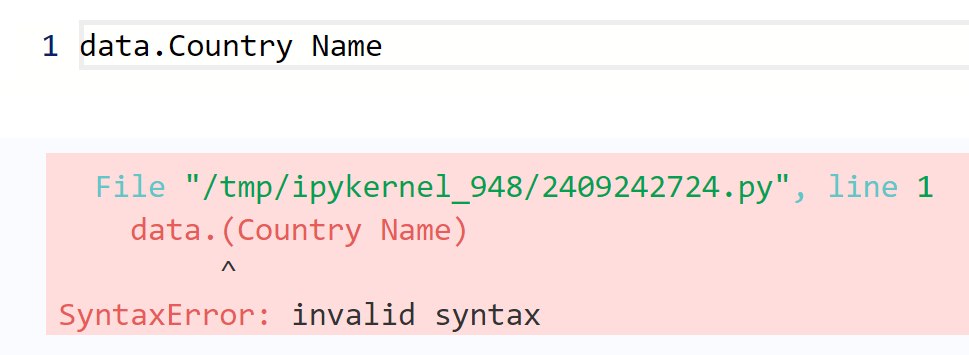那就改一下列名呗！

``````data2 = data
data2.columns = data2.columns.str.strip('Country ')
# print(data2.columns)
print(data2.Name)
``````

这样语法问题就解决了。

``````#请写出1960年GDP最高的国家
data_no_World = data[data.Name != 'World']
max_id = data_no_World['1960'].idxmax()
max_name = data_no_World.iloc[max_id].Name

print(max_name)
``````

输出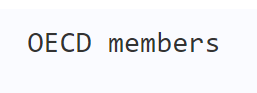代码可以挤一挤写成这样：（但是阅读起来就非常困难了，不建议这样写）

``````#请写出1960年GDP最高的国家
name_max = data.iloc[data[data.Name != 'World']['1960'].idxmax()]['Name']
print(name_max)
``````

但是我后来发现，这个表格中很多都是多国家组成的组织，可能也就不需要去考虑得到的最大GDP是不是一个国家的了。

最终代码：

``````name_max = data.iloc[data['1960'].idxmax()]['Name']
print(name_max)
# 输出：World
``````

### 注意：Dataframe用来赋值返回的是一个引用

Dataframe 用来赋值返回的是引用，而不是建立的一个新的副本。

``````data2 = data
data2.columns = data2.columns.str.strip('Country ')
print(data2.columns)
print(data.columns)
``````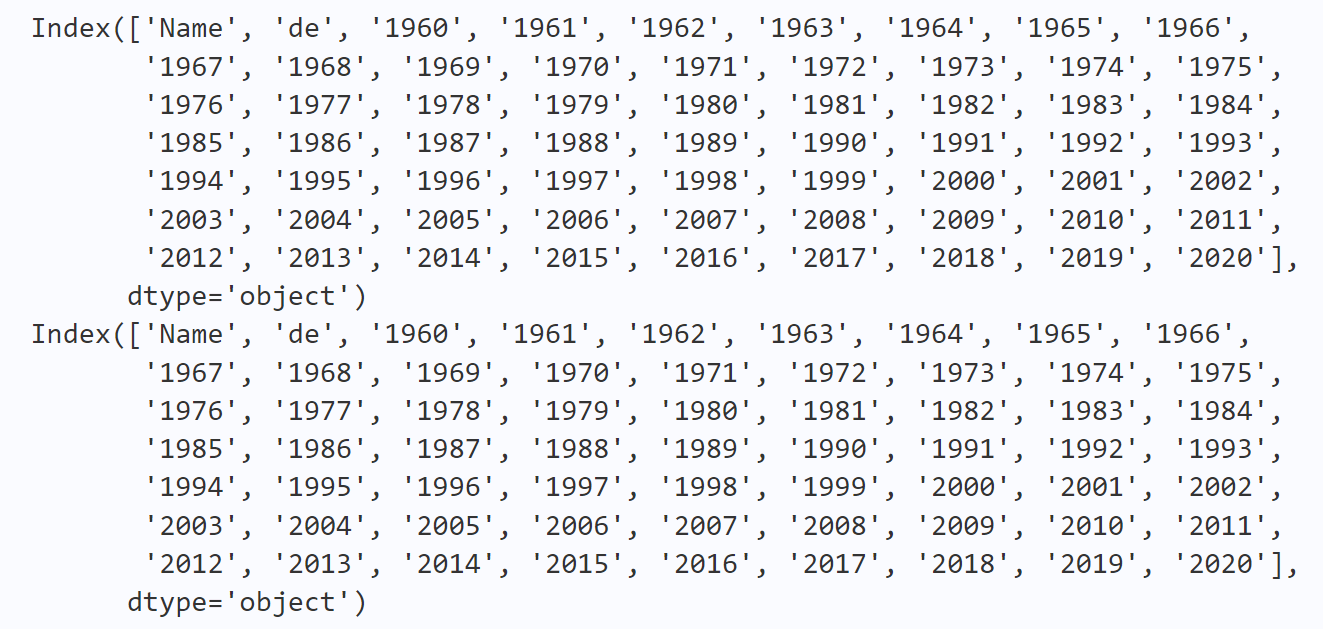## 2、求多个年份GDP前三的国家

写个函数用来求某一年GDP前三的国家，然后多次调用它。

函数逻辑：

• 先求GDP最大的国家，保存下名字
• 排除掉最大的国家后，找GDP最大的国家，保存下名字
• 重复上一步
• 返回：GDP前三国家名的列表
• 如何去掉一个国家？

进行了一些尝试但程序都还是没有跑起来，最后还是用了在索引中加判断进行筛选的方法。代码终于能跑了，不过还是尽量少写这样的垃圾代码😅。

最终代码：

``````#请写出1960、1979、1980、1990、2000、2010、2020年GDP前三的国家
def gdp_top3(year):
# 代码可以再优化
namesTop3 = []
nameMax = data.iloc[data[year].idxmax()]['Name']
namesTop3.append(nameMax)

nameMax = data.iloc[data[year][data.Name != nameMax].idxmax()]['Name']
namesTop3.append(nameMax)

nameMax = data.iloc[data[year][data.Name != namesTop3][data.Name != namesTop3].idxmax()]['Name']
namesTop3.append(nameMax)

return namesTop3

years = [1960, 1979, 1980, 1990, 2000, 2010, 2020]

for i in years:
top3Name = gdp_top3(str(i))
print(i, ': ', top3Name)
``````

算法效率

求一列数中值最大的三个，应该可以在一次遍历中完成。但是上面的代码每次求 top3 都遍历了三次，效率是比较低的。

## 3、选择几个你感兴趣的国家，画合适的图表示他们在60年间的GDP变化

可能需要用到的知识：

• 有多个国家，可能用到多张图在一张画布上的排版
• 描述GDP变化，可以试试刚学的直方图
• 需要在表格按行取出数据，并划分为国家名、GDP两部分
• 流程：

• 1、选择国家，不如就选前四个（方便，先学会操作再说）
• ### 问题1：‘list’ object has no attribute ‘plot’

用的下面代码的格式，前面的数据的类型是 list 列表。

``````titanic_data['Age'].plot.hist(bins=12, title='Age distribution',color=['#00BFFF'])
``````

`error:'list' object has no attribute 'plot'`，是需要 Dataframe 类型吗？那就用这个类型叭！

### 问题2：画直方图，发现x、y轴反了

画出直方图的横、纵轴反了。

``````ctGDP = data.iloc[2:]
ctName = data.iloc[0:1]
ctGDP.plot.hist(bins=60, title=ctName, color=['#00BFFF'])
``````猜测：可能不适合用直方图，不如试试折线图叭！

``````ctGDP = data.iloc[2:].tolist()
x = list(range(1960, 2021))
print(x, type(x))
plt.plot(x, ctGDP)
``````### 问题3：matplotlib绘图，坐标轴无法显示中文

matplotlib 绘图坐标轴无法显示中文，网上搜了一些方法，并没能成功解决。最常见的解决方法是插入以下代码：

``````import matplotlib
matplotlib.rcParams['font.sans-serif'] = ['SimHei']     # 显示中文
``````

不知道是不是我使用的是 Al Studiou 的在线 notebook 的原因，运行这段代码会报错，大意应该是找不到`SimHei`这个字体。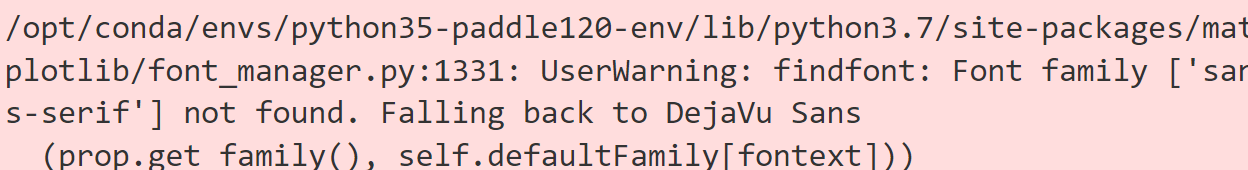那我只好暂时先用着英文的轴标签了。

最终代码与运行效果

``````#请选择几个你感兴趣的国家，画合适的图表示他们在60年间的GDP变化
import matplotlib.pyplot as plt
from matplotlib.gridspec import GridSpec

plt.figure(figsize=(20, 3))
the_grid = GridSpec(1, 4)

x = list(range(1960, 2021))
where = [(0, 0), (0, 1), (0, 2), (0, 3)]

for i in range(4):
ctGDP = data.iloc[i][2:].tolist()
ctName = data.iloc[i][0:2].tolist()
plt.subplot(the_grid[where[i]])
plt.plot(x, ctGDP)
plt.xlabel('years')
plt.ylabel('GDP')
plt.title(ctName)
``````## 4、自选问题：这60年间，GDP增长率最大的国家（或组织）是哪个？

需要用到

• 取某个国家某年的GDP值
• 流程

• 1、分别取 1960 和 2020 年国家的GDP
• 2、求出增长率
• 3、找增长率最大的国家
• 源代码与运行结果

``````#请写出一个你自己感兴趣的问题，并进行探索
#问题：这60年间，GDP增长前三的国家（或组织）是哪些？
GDP1 = data['1960']
GDP2 = data['2020']
rise = GDP2 - GDP1
riseRate = rise / GDP1
index_max = riseRate.idxmax()
name_max = data.iloc[index_max]['Name']
print('这60年间GDP增长率最大的国家:', name_max)
print('GDP增长倍数:', riseRate[index_max])
print('1960年GDP：', GDP1[index_max])
print('2020年GDP：', GDP2[index_max])
``````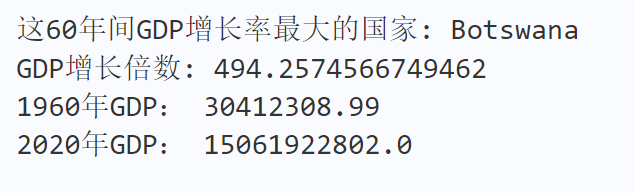感觉学到这里，一些操作慢慢地就熟练起来了。

# 🧭 经验总结

1、有时 debug 就一条思路走到了黑，为了解决一个问题，又引出个问题，问题套问题，最后都忘记了最初是要干什么不要陷入了问题的网罗！